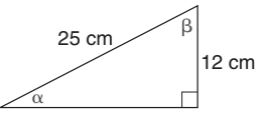Chapter 11.1, Problem 21EElementary Geometry For College St...

7th Edition
Alexander + 2 others
ISBN: 9781337614085

Solutions

Chapter
SectionElementary Geometry For College St...

7th Edition
Alexander + 2 others
ISBN: 9781337614085
Textbook Problem

In Exercises 21 to 26, find the measures of the angles named to the nearest degree.To determine

To measure:

The angle named to the nearest degree.

Explanation

In a right angle triangle,

1) The side PQ, which is opposite to the right angle PRQ is called the hypotenuse.

(The hypotenuse is the longest side of the right triangle.)

2) The side RQ is called the adjacent side of angle θ.

3) The side PR is called the opposite side of angle θ.

Formula used:

sinθ=oppositehypotenuse

Given:

By using Pythagoras theorem, that is

c2=a2+b2

Let b be the adjacent side of the triangle.

From, the diagram we have c=25 and a=12

252=122+b2625=144+b2b2=625144b2=481b=481b=21.9

For angle α, the opposite side is 12 and hypotenuse side is 25.

Then,

sin α=oppositehypotenusesin α=1225sin α=0

Still sussing out bartleby?

Check out a sample textbook solution.

See a sample solution

The Solution to Your Study Problems

Bartleby provides explanations to thousands of textbook problems written by our experts, many with advanced degrees!

Get Started

The radius of convergence of is: 1 3 ∞

Study Guide for Stewart's Multivariable Calculus, 8th

In Exercises 1926, pivot the system about the circled element. [2431|82]

Finite Mathematics for the Managerial, Life, and Social Sciences

True or False: The function whose graph is given at the right is a probability density function.

Study Guide for Stewart's Single Variable Calculus: Early Transcendentals, 8th

Solve by graphing: {x216+y212=1x2y23=1.

College Algebra (MindTap Course List)